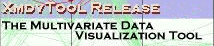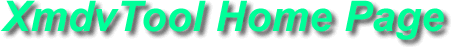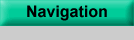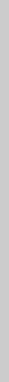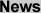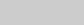VDA 2016 Best Paper Award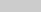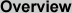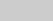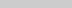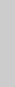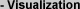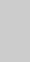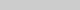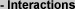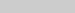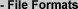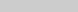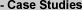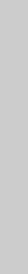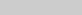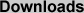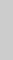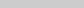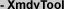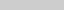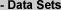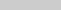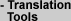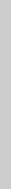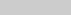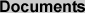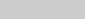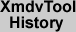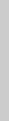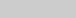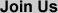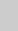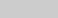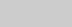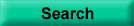powered by FreeFind

XmdvTool File Formats

1. Flat (Non-Hierarchical) Format

All versions of XmdvTool support a flat version of the data set, consisting of ASCII text alphanumeric fields separated by blanks or newlines. The data, structured as rows and columns of integer or real values, is preceded by a simple header, giving data dimensions, labels, and ranges of values for each dimension. Each dimension also has a cardinality, which indicates how many bins will be used for dimensional stacking (if you are uncertain how to specify this, just set it at a relatively small integer, say 2-4).

The basic flat data format, normally given a .okc extension, is structured as follows.

Int_N_number_of_dimensions Int_M_number_of_datapoints
String_fieldName_dimension1
String_fieldName_dimension2
...
String_fieldName_dimensionN
Float_minimum_dimension1 Float_maximum_dimension1 Int_cardinality_dimension1_in_dimstack
Float_minimum_dimension2 Float_maximum_dimension2 Int_cardinality_dimension2_in_dimstack
...
Float_minimum_dimensionN Float_maximum_dimensionN Int_cardinality_dimensionN_in_dimstack
Float_value_dimension1_datapoint1 Float_value_dimension2_datapoint1 ... Float_value_dimensionN_datapoint1
Float_value_dimension1_datapoint2 Float_value_dimension2_datapoint2 ... Float_value_dimensionN_datapoint2
...
Float_value_dimension1_datapointM Float_value_dimension2_datapointM ... Float_value_dimensionN_datapointM

Note: Blank is not allowed to be used in the fieldNames. If you use "(" in the fieldNames, system will omit all the contents after it in the same line.

2. Hierarchical Data Format

In XmdvTool4.0, we introduced the concept of hierarchical visualization of multivariate data, and provided tools for creating hierarchical clusters from flat data files. In fact, there are many techniques for creating such hierarchies, either through the natural structure of the data (e.g., a file system), binning of dimensions (e.g., data cube methods), and algorithmic techniques (clustering, partitioning). The format required to use the hierarchical approaches should be a file with a .cf extension, should be put in the same directories as their coresponding .okc files.

Formats of a .cf file:
Int_L_number_of_nodes Int_N_number_of_dimensions
Int_id_node1 Int_parent_node1 Int_entries_node1 Float_sx1_node1 ... Float_sxN_node1 Float_sqrtRadius_node1
Int_id_node2 Int_parent_node2 Int_entries_node2 Float_sx1_node2 ... Float_sxN_node2 Float_sqrtRadius_node2
...
Int_id_nodeL Int_parent_nodeL Int_entries_nodeL Float_sx1_nodeL ... Float_sxN_nodeL Float_sqrtRadius_nodeL

In XmdvTool4.1, we refined this format to include more aggregation information. Specifically, rather than assigning a single value to a cluster (radius, as was used in the Birch Clustering Algorithm), we specify extents for each dimension. Thus the data file format for using the hierarchical approaches have a .cg extension and should be put in the same directories as their coresponding .okc file.

Format of a *.cg file:
Int_L_number_of_nodes Int_N_number_of_dimensions
Int_id_node1 Int_parent_node1 Int_entries_node1 Float_sx1_node1 ... Float_sxN_node1 Float_sqrtRadius_node1 Float_max1_node1 ... Float_maxN_node1 Float_min1_node1 ... Float_minN_node1
Int_id_node2 Int_parent_node2 Int_entries_node2 Float_sx1_node2 ... Float_sxN_node2 Float_sqrtRadius_node2 Float_max1_node1 ... Float_maxN_node1 Float_min1_node1 ... Float_minN_node1
...
Int_id_nodeL Int_parent_nodeL Int_entries_nodeL Float_sx1_nodeL ... Float_sxN_nodeL Float_sqrtRadius_nodeL Float_max1_node1 ... Float_maxN_node1 Float_min1_node1 ... Float_minN_node1

You can find examples of .okc, .cf and .cg files in the download page.